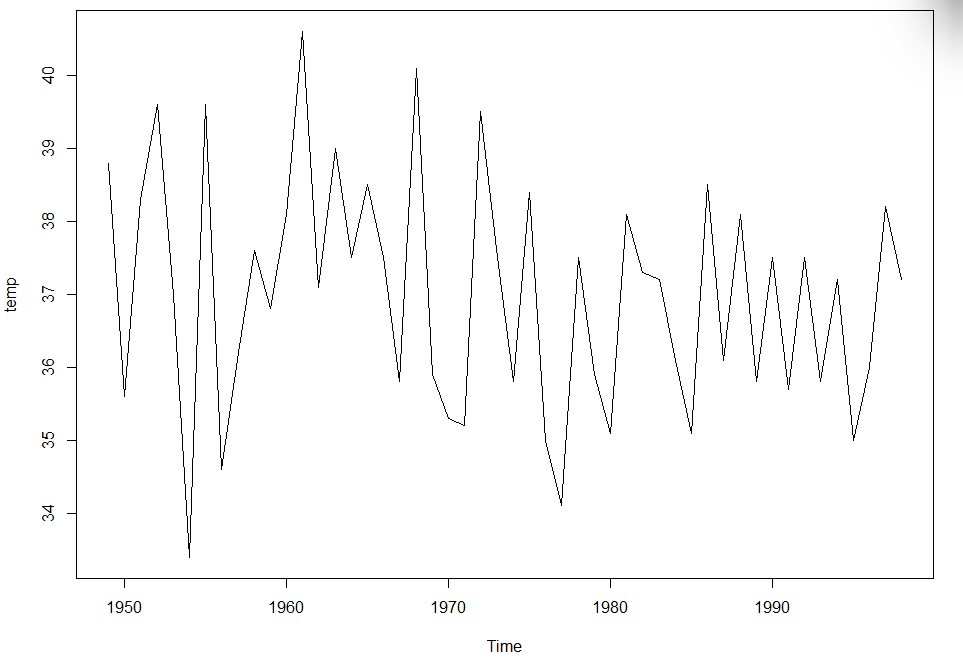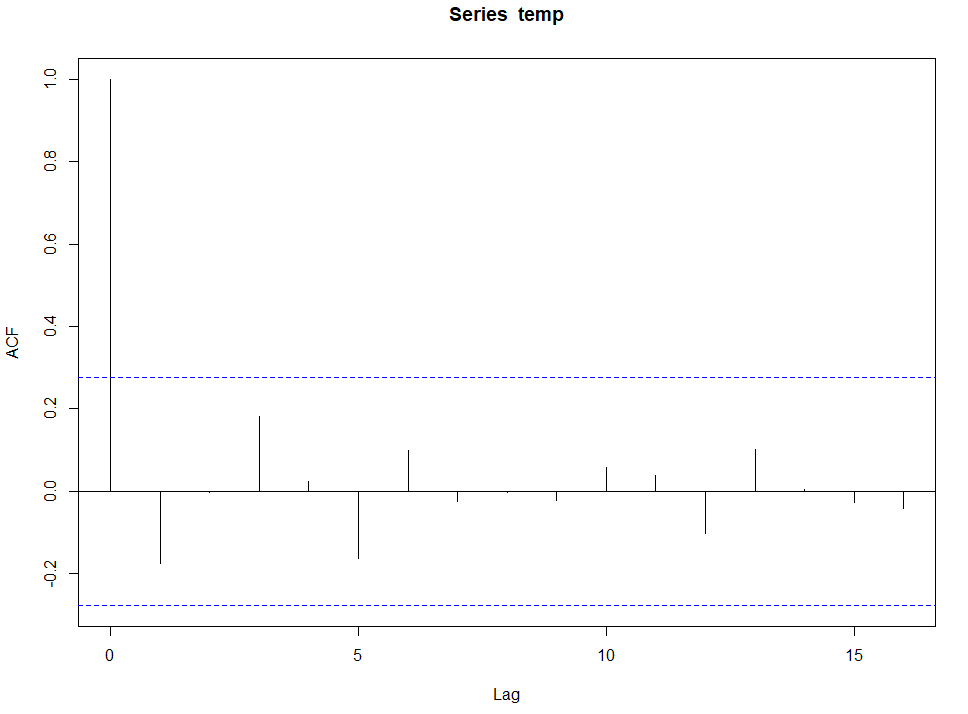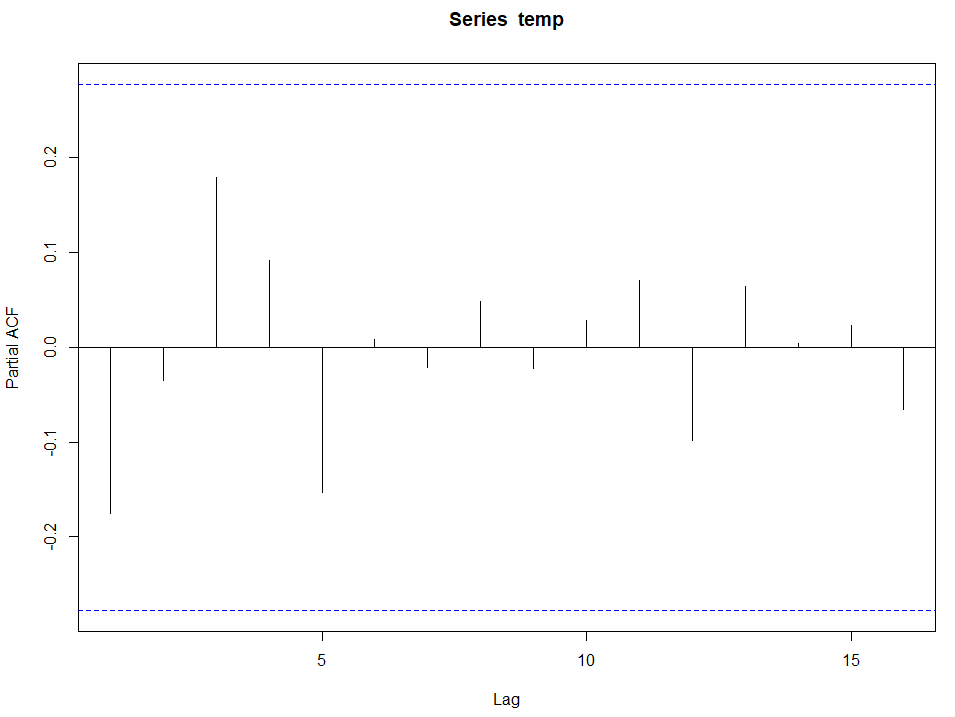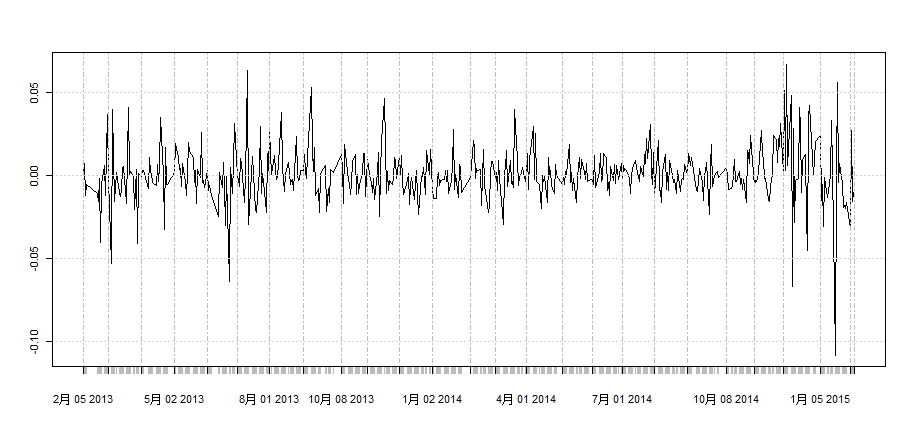• r语言画时序图
千次阅读
2022-03-04 09:33:35

这学期的《应用时间序列分析》、《R语言》、《统计案例分析》都需要使用R语言，故将课程中学到的代码以及相关补充代码分享出来，一方面促进自身学习的积极主动性，另一方面或许可以给学习R语言的同学们提供一点点帮助。

rm(list=ls())
install.packages("tseries")
install.packages("astsa")
install.packages("zoo")#插值
library(tseries)
library(astsa)
library(zoo)

##时间序列数据展示——自输
#函数ts

price = c(101,82,66,35,31,7,9,4)
price = ts(price,start = c(2005,1),frequency = 12 )
price
##时间序列数据展示——scan

price = scan()#填完数据之后要回车一下，得到Read n items
price = ts(price,start = c(2005,1),frequency = 12 )
price

##输入数据
data = read.table(".csv",sep = ",",header = T)
data = read.table(".txt",sep = "\t")

##缺失值插值
a = c(1,3,6,9,10,24,79)
a = NA
a

#线性插值
y1 = na.approx(a)
y1

#样条插值
y2 = na.spline(a)
y2

##数据读出
write.table(data.frame(),file = ".csv",sep = ",",row.names = F)

##描述时间序列数据
#只有一个时间序列
plot(data)
#比较两个时间序列的关系——一个变量
par(mfrow = c(2,1))
plot(data1,main = "")
plot(data2,main = "")
#比较两个时间序列的关系——两个变量
par(mfrow = c(2,1))
ts.plot(data1[1:4],col = 1:4,ylab = "",main = "")
ts.plot(data2[6:9],col = 1:4,ylab = "",main = "")

###序列平稳性地图检验法：
##时序图

data
#将数据变成时间序列数据
data = ts(data,start = ,end = ,frequency = )#一般情况下写个start和frequency就可以了
#画图
plot(data,type = "o",pch = "23",lty = 4,lwd = 2,xlim = c(),col = 'blue',main = '',ylab = '',xlab='')
abline(v = c(x),h = c(y),lty = 2)
#检验序列平稳性1：平稳时间序列时序图，在一个常数值附近随机波动，且波动范围有界

##自相关图
acf(data,lag = n)
#检验序列平稳性2：自相关系数随着延迟期数k的增加，平稳序列的自相关系数会很快地衰减到零。r语言
更多相关内容
• p<-ts(data$水位,start=c(1860),frequency=1) #Draw a sequence diagram plot(p,type = "o") #Draw autocorrelation diagram acf(p,lag.max = 25) #Check whether the sequence is ...效果 运行结果 Box-Ljun p<-ts(data$水位,start=c(1860),frequency=1)

#Draw a sequence diagram
plot(p,type = "o")

#Draw autocorrelation diagram
acf(p,lag.max = 25)

#Check whether the sequence is a pure random sequence
Box.test(p,lag = 12,type = "Ljung-Box")



效果图运行结果
Box-Ljung test

data: p
X-squared = 329.2, df = 12, p-value < 2.2e-16

展开全文r语言
• 画时序图 install.packages("readxl") library(readxl) te <- read_xls("D:/data/Rdata/tsdata.xls") temp <- ts(te$温度,start=1949) plot(temp) 本来用的函数是read.table()，但是一会儿中文乱码的，一会... # 画时序图 install.packages("readxl") library(readxl) te <- read_xls("D:/data/Rdata/tsdata.xls") temp <- ts(te$温度,start=1949)
plot(temp)


本来用的函数是read.table()，但是一会儿中文乱码的，一会儿报错的，就选择了readxl库去读取xls文件。
ts函数可以生成类型是Time Sreies的时序数据，然后直接plot就可以画出时序图，横坐标默认是Time，纵坐标是时序数据的变量名。# 画相关图

acf(temp)# 画自相关图

pacf(temp)acfpacf函数中除了变量名，都还有一个参数：延迟阶数lag，如果用户不特殊指定的话，系统会根据序列长度自动指定延迟阶数。

展开全文r语言
• ## 利用R语言画简单时间序列图

万次阅读 多人点赞 2017-08-27 14:57:03
所以利用R语言画时间序列的一个关键步骤就是将读取的数据转变为时间序列格式， 下面是一个简单的程序： # 读取数据， 首先将excel 格式的转化为 csv 格式 再读取 h (file = "D:/data/50etfx1.csv",sep = ",",...

R 语言无法自动将读取的数据转化为时间序列格式，

所以利用R语言画时间序列图的一个关键步骤就是将读取的数据转变为时间序列格式，

下面是一个简单的程序：

 #  读取数据， 首先将excel 格式的转化为 csv 格式 再读取
h <- read.table(file = "D:/data/50etfx1.csv",sep = ",",header = T)
#  将数据格式转化为时间序列格式
library(xts)  # 加载需要的包
hh <- xts(h$x1, as.Date(h$time, format='%Y/%m/%d'))
#  画图
# 指定图的长宽 或者 用命令  plot(hh)  直接画
win.graph(width = 9.5,height = 4.5,pointsize = 8)
plot(hh,type = 'l',main=' ')
效果如下：示例程序所用的数据格式如下所示：

time                   x1
2013/2/5          0.000000
2013/2/6          0.007236
2013/2/7          -0.011708
2013/2/8    -0.004989
2013/2/18 -0.010806
2013/2/19 -0.015255
2013/2/20 -0.001583
2013/2/21 -0.040148

。。。。。。。。。。。。

2015/1/29 -0.019893
2015/1/30 -0.016171
2015/2/2          -0.031637
2015/2/3          0.027171
2015/2/4         -0.015722
2015/2/5         -0.010018
2015/2/6         -0.012613

展开全文R语言 数据读取
• ## 利用R语言绘制时间序列图

万次阅读 多人点赞 2020-06-26 22:37:57
数据 GDP.csv文件,存储1879~2019年河南省GDP数据 绘图 # 读取数据， 首先将excel 格式的转化为 csv 格式 再读取 h <- read.table(file = "C:/Users/PYY/Desktop/GDP.csv",sep = ",",header = T) ...R语言 时序图
• R语言时间序列（time series）分析实战：时序数据加载、绘制时间序列 目录 R语言时间序列（time series）分析实战：时序数据加载、绘制时间序列 #数据集 #加载时间序列数据 #绘制时间序列 #数据...r语言 数据挖掘 机器学习 自然语言处理 人工智能
• 数据是这样的是一个疾病的发病率，我想画时序图下边这样的 我画出来的是下图，是自动生成的横坐标，显示的是1，2，一直到120，我是想问如何才能画出上图呢，让我的横坐标显示date那一栏的数据 在网上搜了，用了 plot...r语言
• 主要内容：R语言绘制时序图时，自定义设置x轴时间刻度为天/周/月 数据介绍：北京市2020年5月1日至2020年7月31日空气环境质量指数 目录plot函数绘制ggplot函数绘制 plot函数绘制 主要思想：删除原有标签，自定义新的...r语言 ggplot2
• e<-rnorm(100) x1<-filter(e,filter = c(1,-0.5),method = "convolution",circular = T) plot(x1) acf(x1) pacf(x1)r语言
• ## r语言时间序列图_R中的时间序列图

万次阅读 多人点赞 2020-07-18 23:54:52
r语言时间序列In this tutorial, we’ll be going over how to create time series plots in R. Time series data refers to data points that represent a particular variable changing over different points ...python 大数据 机器学习 java
• R语言用具体时间做x轴绘图，使用as.POSIXct（）函数r语言
• 效果如下 library(igraph) # Create data set.seed(1) data <- matrix(sample(0:1, 100, replace=TRUE, prob=c(0.8,0.2)), nc=10) network <- graph_from_adjacency_matrix(data , mode='undirected', diag=...r语言
• R语言使用ggplot2包可视化时间序列折线、同时可视化多个时间序列的折线、自定义折线的颜色数据挖掘 r语言 人工智能 机器学习
• R的可视化绘图中的图形坐标轴的间距刻度设置、图形上添加数字标签、图形中给点添加文字标签、图形添加水平虚线、绘制函数轨迹曲线、图形加外框并注解该含义、坐标轴上单独加函数：y = 1/x、坐标轴的距离位置、增加...
• 为啥要画图 复杂问题、复杂系统的根本解决之道，归根结底就是两个字：分解。 对问题的分析、分解过程，借助图形化的表达，可以达到“一...所以，流程制作的关键，不是在于熟悉和熟练使用几种图形元素，而更在于，真时序图 架构图 拓扑图 部署图
•r语言
• R语言ggplot2可视化：使用ggplot2绘制按时间顺序排列的时间线图（chronological timeline plot）r语言 机器学习 人工智能 数据挖掘
• R语言绘制时间序列的自相关函数：使用acf函数可视化时间序列数据的自相关系数
• 并绘制收益率的时序图 close = data\$close return * diff(log(close)) cls2 (return,date) plot(cls2) （3）对收益率数据进行描述性统计分析 data_outline (x){ n (x) m (x) v (x) s (x) me (x) cv *s/m #变异系数 ...r语言
• ## R语言：时间序列

千次阅读 2020-12-08 18:12:37
根据观察时序图以及白噪声检验Box.test()，进行平稳性判别的检验 若得到平稳的非白噪声序列，则进行模式识别 自相关图和非自相关图，根据两图的结尾性和拖尾性进行AR、MA、ARMA的模式识别 对识别后模式中的位置...r语言
• R绘制时间序列图形（time series） ...# ggfortify包的autoplot函数可以自动绘制时间序列对象对应的时序图； ## From Timeseries object (ts) library(ggplot2) install.packages('ggfortify')R ggplot2 数据挖掘 机器学习
• ## R语言之plot()画图

千次阅读 2020-12-09 09:51:41
参数取值 描述 type = “p” 点 type = “l” 线 type = “b” 点连线 type =“o” 线穿过点 type =“h” 悬垂线 type = “s” 阶梯线 操作示例 # 时序图 yield (15.2, 16.9, 15.3, 14.9, 15.7, 15.1, 16.7) yield ...r语言
• 时间序列R语言实操1 运用到openair中的calendarPlot calendarPlot( mydata, pollutant = "nox", year = 2003, month = 1:12, type = "default", annotate = "date", statistic = "mean", cols = "heat", ...r语言 时序模型
• R语言点线结构参数 参数type的取值及定义 参数取值 描述 type='p' 点 type='l' 线 type='b' 点连线 type='o' 线穿过点 type='h' 悬垂线 type='s' 阶梯线r语言
• R语言相对于python在做统计分析是十分方便的软件，时间序列分析在数理统计理论方面很有支撑，解释性也很强，理论已经很成熟，不了解的小伙伴可以去搜下相关课程。 这里记录一下简单的R语言做时间序列分析的流程。 ...r语言
• 目录0引言1、customLayout包1.1 主要函数介绍 ...在之前使用R语言拼接图片，一般的图形用的是par函数，ggplot2的拼接使用的是gridExtra包里的grid.arrange函数。 1、customLayout包 1.1 主要函数介绍 ...R语言可视化 图片拼接
• @R语言进行Box-Cox变换 R语言进行Box-Cox变换 Box-Cox变换是Box和Cox在1964年提出的一种广义幂变换方法，是统计建模中常用的一种数据变换，用于连续的响应变量不满足正态分布的情况。Box-Cox变换的主要特点是引入一...r语言
• 2、R语言 n=1000 x=sample(x=c(-1,1),n,TRUE) s=cumsum(x)#对x进行累加求和 plot(ts(s))#ts()强制成时间序列 其中，将时间边长，可以明显看出ts(s)的范围更加广泛： 查看自相关函数，可以看出随机游走模型本身...时序模型 r语言...